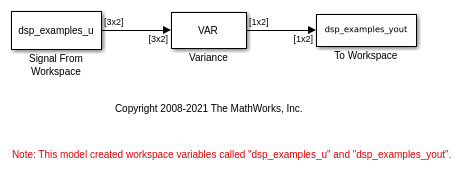# Compute the Variance

Compute the variance of a 3-by-2 matrix input `dsp_examples_u` using the Variance block.

Open and run the model. In the Variance block dialog, set the Find the variance value over parameter to `Each column`.The Variance block processes the input as a two-channel signal with a frame size of three, and outputs the variance value over each frame of data along both the channels.

```Data Input 6 1 1 3 3 9 -7 2 2 4 5 1 8 6 0 2 -1 5 -3 0 2 4 1 17 Variance Values 6.3333 17.3333 39.0000 2.3333 24.3333 4.3333 7.0000 79.0000 0 0 0 0 ```